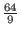Next: Overview of Shor's Algorithm Up: Quantum Algorithms Previous: Introduction to Shor's Algorithm   Contents

## Motivation for Shor's Algorithm

The algorithm was viewed as important because the difficulty of factoring large numbers is relied upon for many cryptography systems. If an efficient method of factoring large numbers is implemented most of the many encryption schemes would be next to worthless to protect their data from prying eyes. While it has not been proven that factoring large numbers can not be archived on a classical computer in polynomial time, as of 2015 the fastest algorithm publicly available for factoring large number runs in O(exp(n1/3(log n)2/3), operations where n is the number of bits used to represent the number: this runtime exceeds polynomial time. In contrast Shor's algorithm runs in O((log n)2*loglog n) on a quantum computer, and then must perform O(log n) steps of post processing on a classical computer. Overall then this time is polynomial. This discovery propelled the study of quantum computing forward, as such an algorithm is much sought after. (Shor)Next: Overview of Shor's Algorithm Up: Quantum Algorithms Previous: Introduction to Shor's Algorithm   Contents
Matthew Hayward - Quantum Computing and Shor's Algorithm GitHub Repository